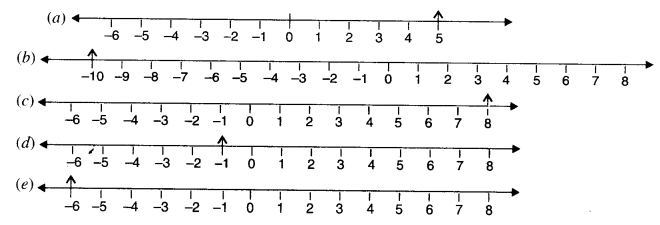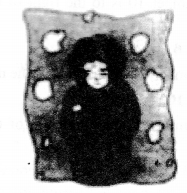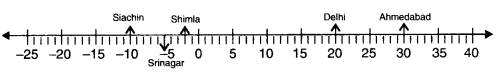NCERT Solutions for Class 6 Maths Chapter 6 Integers Ex 6.1 are part of NCERT Solutions for Class 6 Maths. Here we have given NCERT Solutions for Class 6 Maths Chapter 6 Integers Ex 6.1.

 Board CBSE Textbook NCERT Class Class 6 Subject Maths Chapter Chapter 6 Chapter Name Integers Exercise Ex 6.1 Number of Questions Solved 10 Category NCERT Solutions

## NCERT Solutions for Class 6 Maths Chapter 6 Integers Ex 6.1

Question 1.
Write opposite of the following:
(a) Increase in weight
(b) 30 km north
(c) 326 BC
(d) Loss of Rs. 700
(e) 100 m above sea level.
Solution.
(a) Decrease in weight
(b) 30 km south
(d) Gain of Rs. 700
(e) 100 m below sea level

Question 2.
Represent the following numbers as integers with appropriate signs.
(a) An airplane is flying at a height two thousand meters above the ground.
(b) A submarine is moving at depth, eight hundred meters below the sea level.
(c) A deposit of rupees two hundred.
(d) Withdrawal of rupees seven hundred.
Solution.
(a) + 2000
(b) – 800
(c) + 200
(d) – 700.

Question 3.
Represent the following numbers on a number line:

(a) + 5
(b) -10
(c) + 8
(d) -7
Solution.Question 4.
Adjacent figure is a vertical number line, representing integers. Observe it and locate the following points :
(a) If point D is + 8, then which point is – 8?
(b) Is point G a negative integer or a positive integer?
(c) Write integers for points B and E.
(d) Which point marked on this number line has the least value?
(e) Arrange all the points in p-decreasing order of value.
Solution.(a) F
(b) negative integer
(c) B → + 4, E → 10
(d) E
(e) D, C, B, A, O, H, G, F, E.

Question 5.
Following is the list of temperatures of five places in India, on a particular day of the year.
Solution.

 Place Temperature Siachin 10°C below 0°C …………. Shimla 2°C below 0°C ………… Ahmedabad 30°C above 0°C …………. Delhi 20°C above 0°C ……….. Srinagar 5°C below 0°C ………….(a)
Write the temperature of these places in the form of integers in the blank column.
(b) Following is the number line representing the temperature in degree Celsius.
Plot the name of the city against its temperature.
(c) Which is the coolest place?
(d) Write the names of the places whose temperature are above 10°C.
Solution.
(a)

 Place Temperature Siachin 10°C below 0°C – 10°C Shimla 2°C below 0°C – 2°C Ahmedabad 30°C above 0°C + 30°C Delhi 20°C above 0°C + 20°C Srinagar 5°C below 0°C – 5°C

(b)(c) Siachin is the coolest place.

Question 6.
In each of the following pairs, which number is to the right of the other on the number line?
(a) 2, 9
(b) -3,-8
(c) 0,-1
(d) – 11, 10
(e) -6,6
(f) 1,- 100
Solution.
(a) 2, 9
The number 9 is to the right of the number 2.
(b) -3,-8
The number – 3 is to the right of the number -8.
(c) 0,-1
The number 0 is to the right of the number – 1.
(d) – 11,10
The number 10 is to the right of the number -11.
(e) -6, 6
The number 6 is to the right of the number – 6.
(f) 1, -100
The number 1 is to the right of the number – 100.

Question 7.
Write all the integers between the given pairs (write them in the increasing order)
(a) 0 and – 7
(b) -4 and 4
(c) – 8 and -15
(d) – 30 and – 23.
Solution.
(a) 0 and – 7
The integers between 0 and – 7 in increasing order are – 6, – 5, – 4, – 3, – 2 and – 1.
(b) – 4 and 4
The integers between – 4 and 4 in increasing order are – 3, – 2, – 1, 0, 1, 2 and 3.
(c) – 8 and – 15
The integers between – 8 and – 15 in increasing order are – 14, – 13, – 12, – 11, – 10 and – 9.
(d) – 30 and – 23
The integers between – 30 and – 23 in increasing order are – 29, – 28, – 27, – 26, – 25 and – 24.

Question 8.
(a) Write four negative integers greater than – 20.
(b) Write four negative integers less than -10.
Solution.
(a) Four negative integers greater than 20 are – 19, – 18, – 17 and – 16.
(b) Four negative integers less than – 10 and 11,- 12,- 13 and -14

Question 9.
For the following statements write True (T) or False (F). If the statement is false, correct the state­ment.
(a)
-8 is to the right of- 50 on a number line.
(b) – 100 is to the right of – 50 on a number line.
(c) A smallest negative integer is – 1.
(d) – 26 is larger than -25
Solution.
(a) True (T)
(b) False (F); – 100 is to the left of – 50 on a number line.
(c) False (F); Greatest negative integer is – 1.
(d) False (F); – 26 is smaller than – 25.

Question 10.
Draw a number line and answer the following:
(a) Which number will we reach if we move 4 numbers to the right of-2?
(b) Which number will we reach if we move 5 numbers to the left of 1?
(c) If we are at -8 on the number line, in which direction should we move to reach -13?
(d) If we are at-6 on the number line, in which direction should we move to reach -l?
Solution.
(a) We will reach number 2.
(b) We will reach the number – 4.
(c) We should move in the left direction.
(d) We should move in the right direction

We hope the NCERT Solutions for Class 6 Maths Chapter 6 Integers Ex 6.1 help you. If you have any query regarding NCERT Solutions for Class 6 Maths Chapter 6 Integers Ex 6.1, drop a comment below and we will get back to you at the earliest.